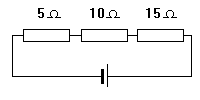Home > Electronics Tutorials > Direct Current (DC) Theory > Resistors in Series Tutorial

Direct Current (DC) Theory - Electronics Teaching

# Resistors in Series TutorialResistors in series are connected in line.

The same current flows through them all.

The total opposition to the flow of current is called the EQUIVALENT resistance.

To find the value of the equivalent resistance we simply add the values.

In this case it is 30 ohms.

Note that, as a quick check on calculations, the value of the equivalent resistance is always higher than the value of the highest value resistance.

If these resistors were connected across a 30 Volt battery then Ohms Law says 1 amp would flow.